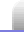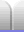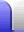# Factorising Tricks

### Table of Factorising Tricks

How do we know if a number is divisible by a particular smaller number? In other words, does a number have a particular smaller number as a factor? It would certainly make factorisation easier.

The most straightforward way is recognising the number from our times tables. There are also other ways we can break numbers down to help spot particular factors.

 Most useful (and easiest to remember) Divisible by (primes are bold) Trick2 Number is even.3 The sum of the number's digits adds to a multiple of 3. (See examples below.) 4 The number is even and halving the last two digits also gives an even number. OR take the last two digits and knock multiples of 20 off to get a multiple of 4 that's ≤16. OR twice the tens digit plus the ones digit is a multiple of 4. (In other words, for multiples of 4, if the ones digit is 0, 4 or 8 then the tens digit is even, if the ones digit is 2 or 6 then the tens digit is odd.)5 Number ends in 5 or 0.6 Number is divisible by 2 and 3. In other words, is even and its digits adds to a multiple of 3. 7 Subtracting 2 times the last digit from the rest is a multiple of 7. OR adding 5 times the last digit to the rest is a multiple of 7. 8 Halving the number twice in a row still gives an even number. OR knock multiples of 200 off until it's less than 200, then knock multiples of 40 off until the number is less than 40 or you recognise a multiple of 8 from your times tables. OR If the hundreds digit is even, the last two digits will be divisible by 8 (use times tables, subtract known multiples of 8 [eg, 40], etc). If the hundreds digit is odd, the {last two digits plus 4} will be divisible by 8.9 The number's digits adds to a multiple of 9 (ie, 3 x 3).10 Number ends in 0. In other words, it's divisible by 5 and it's even.11 For two digit numbers, the two digits are the same. For three digit numbers, the sum of the first and last digits will be the middle digit. (Note that the middle digit will carry over for some multiples of 11; eg, 627 = 11 x 57.) OR subtracting the last digit from the rest will give a result divisible by 11. OR start with the left-most digit, subtract the next one, add the next one, subtract the next one, etc, and the final result is a multiple of 11. 12 Number is divisible by 3 and 4. 13 The sum of 4 times the last digit added to the rest is a multiple of 13. 15 Number is divisible by 3 and 5. 17 Subtracting 5 times the last digit from the rest will give a multiple of 17. 18 Number is divisible by 2 and 9. 19 Adding 2 times the last digit to the rest will give a multiple of 19.20 Number ends in 0 and the tens digit is even. 21 Number is divisible by 3 and 7. OR subtracting 2 times the last digit from the rest is a multiple of 21. (This is the same rule as 7 except for the result.) 23 Adding 7 times the last digit to the rest will give a multiple of 23.25 Number ends in 25, 50, 75, or 00. 29 Adding 3 times the last digit to the rest will give a multiple of 29. 31 Subtracting 3 times the last digit from the rest will give a multiple of 31.50 Number ends in 50 or 00.100 Number ends in 00. 200 Number ends in 00 and the hundreds digit is even. others For more divisibility rules see divisibility rule.

With tricks for finding factors up to 31, all numbers up to more than 1,000 can be factorised.

Compare the tricks for finding multiples of 19 and 21, and 29 and 31.

### Examples

The trick for figuring out if a number is divisible by 3 is quite handy, and is the basis for figuring out if numbers are divisible by 6 and 9 as well. If we have a big, daunting number, just add the digits together. If we end up with more than one digit in the sum and can't easily recognise it as a multiple of three we can simply do it again. (In other words, the rule can be applied recursively.)

123,456 divisible by 3?
1 + 2 + 3 + 4 + 5 + 6 = 21
2 + 1 = 3

And since 3 is a multiple of 3 we know that 21 is a multiple of 3, which means 123,456 is a multiple of 3. We also know that 123,456 is a multiple of 6 since it's also even. We also know that 654,321 must be divisible by three (but is not a multiple of 6).

Let's try a bigger number. How can we tell at a glance if 123,456,789 is a multiple of 9? If the digits add to a multiple of 9 we will know it's divisible by 9.

123,456,789 divisible by 9?
1 + 2 + 3 + 4 + 5 + 6 + 7 + 8 + 9 = 45
4 + 5 = 9

So yes, 123,456,789 is divisible by 9.

Another way of doing it is that we can simply ignore groups of digits if they add to 9. This is because adding a 9 will not change whether the sum is a multiple of 9 – it'll just be a different multiple of 9.

123,456,789 divisible by 9?
The 9 on the end is, so
Yes if 12,345,678 is divisible by 9
1 + 8 = 9, so
Yes if 234,567 is divisible by 9
2 + 7 = 9, so
Yes if 3,456 is divisible by 9
3 + 6 = 9, so
Yes if 45 is divisible by 9
4 + 5 = 9, so
Yes.

### Application

1. Let's use these tricks to factorise 6,831 – the number of lithium ion cells in the battery of a Tesla electric car.

The first thing I notice is that it's not even, but 8+1 makes 9, which is a multiple of 3, and since 6 and 3 are already multiples of 3, the whole 6,831 must be as well. The next thing I notice is that it's divisible by 9, since 6+3=9 and 8+1=9. So 3 and 3 are factors of 6,831. No calculators, now.

 6,831 = 3 x 2,277 = 3 x 3 x 759 (still divisible by 3) = 3 x 3 x 3 x 253 (3 digits with that pattern, must be divisible by 11) = 3 x 3 x 3 x 11 x 23

Other Tesla batteries include their "85" kWh pack (16 modules of 444 cells; 7,104 total cells) and their "60" kWh pack (14 modules of 384 cells; 5,376 total cells).

2. The sum of three consecutive numbers is always a multiple of three. Using the tricks above can you figure out why?

Hint: n + (n + 1) + (n + 2)Home Astronomy Chemistry Electronics & Computers Mathematics Physics Field Trips Turn on javascript for email link